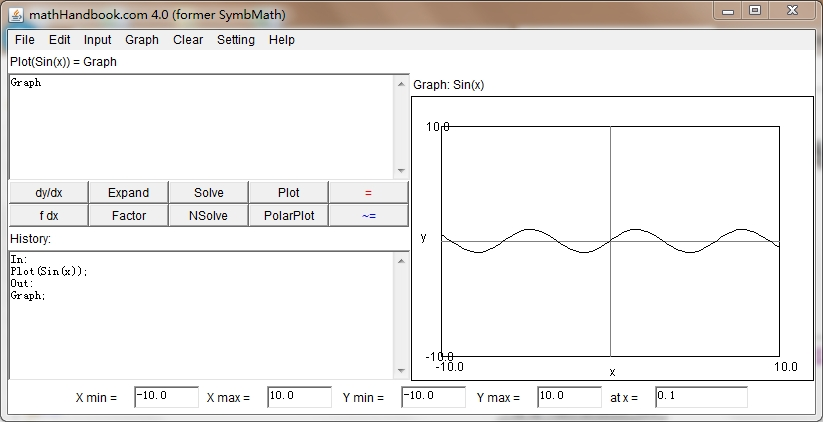﻿﻿ Fractional Calculus Computer Algebra System math software Home | list | math | function | calculator | handbook | help | forum | contact | index | 中文﻿
﻿

convert

convert an expression to a different form.

Calling Sequence Parameters Description Examples Source

Calling Sequence

convert(expr, form)
convert(expr to form)
convert expr to form

Parameters

expr - any expression
form - form name

Description

* The convert function is used to convert an expression from one form to another. Some of the conversions are data-type conversions, for example. Others are form or function conversions, for example convert(sinh(x),exp) yields exp(x)/2-exp(-x)/2 and convert(a*x!, gamma) yields a*gamma(x+1).
* For function conversions, a set of optional arguments to perform the conversion in different manners are described in convert/to_special_function.
* The types of known conversions are (the second argument form must be one of these):

abs
binomial
complex
cos
cosh
d
degree
erf
exp
factorial
float
gamma
int
integral
log
parfrac
polar
poly
polyroot
power
rational
sgn
sin
sinh
sum
trig
trigh
x!

Check the list above for all known types of conversions for more possibilities. Conversions to more advanced mathematical functions are shown below.
* Further information is available under help pages convert/form where form is one of the forms from the above list.
* convert(a to xxx) will call the toxxx() function if the toxxx() function is defined. A user can make custom conversions known to the convert function by defining the toxxx() function.

Examples


Convert can be used to convert numbers between bases.  See the help pages convert/decimal, convert/base, convert/binary, convert/octal, and convert/hex for more information and examples.
>   convert sin(x) to exp
0.5*i*e^((-i)*x)+(-0.5*i)*e^(i*x)	 	(1)

>   convert(pi,degrees)
180degrees 	 (2)

Convert to rational expressions or floating-point numbers. See the help pages convert/float and convert/rational for more information.
>   convert(1.23456,rational)
38583125 	 (3)

>   convert(18,float)
0.125 	 (4)

Convert between units. See the help page convert/units for more information.  Also, see the Units package.
>   convert(22,units,inches,m)
13972500 	 (5)

Convert a rational expression to partial fraction form. See the help page convert/parfrac for more information.
>   f:=(x^3+x)/(x^2-1)
f:=(x^3+x)/(x^2-1)	 (11)

>   convert(f,parfrac)
x+1/(x-1)+1/(x+1)	 (12)

Convert a series to a polynomial by dropping the order term. See the help page convert/poly for more information.
>   s:=series(f,x,4)
s:=-x-2x^3+O(x^5) 	 (13)

>   convert(s,poly)
-2x^3-x 	 (14)

Convert a complex expression to polar coordinates. See the help page convert/polar for more information.
>   convert(12+2i,polar)
polar(1/2*sqrt(2),1/4 pi) 	 (15)

Convert an expression to trigonometric or exponential form, if possible. See the help pages convert/exp and convert/trig for more information.
>   g:=sinh(x)+sin(x)
g:=sinh(x)+sin(x) 	 (16)

>   convert(g,exp)
1/2e^x-1/2e^(-x)-1/2I(e^(I*x)-e^(-I*x)) 	 (17)

> convert gamma(x) to x!
(-1+x)!


﻿